# Banach indicatrix

(diff) ← Older revision | Latest revision (diff) | Newer revision → (diff)

multiplicity function, of a continuous function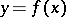,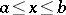An integer-valued function,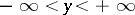, equal to the number of roots of the equation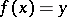. If, for a given value of, this equation has an infinite number of roots, then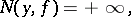and if it has no roots, then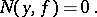The function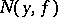was defined by S. Banach  (see also ). He proved that the indicatrixof any continuous function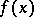in the interval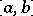is a function of Baire class not higher than 2, and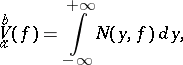(*)

whereis the variation ofon. Thus, equation (*) can be considered as the definition of the variation of a continuous function. The Banach indicatrix is also defined (preserving equation (*)) for functions with discontinuities of the first kind . The concept of a Banach indicatrix was employed to define the variation of functions in several variables , .